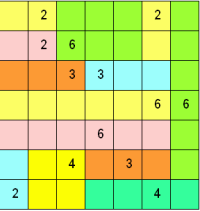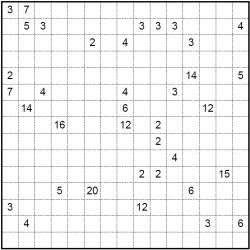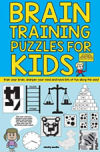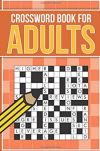Thu, 09 Jul 2020

# Sikaku Puzzles

Sikaku or divide by box is a puzzle that has many names, including shikaku and cell blocks. Another name in English is rectangles. So, those are the names the puzzle goes by, but what exactly is it?

Shikaku is a logic puzzle first and foremost. The game starts with a rectangular grid (typically a square one), which may be of a large number of different sizes from the very small such as 6x6 to full page puzzles that could be as much as 50x50 in dimensions, or even larger. To some extent the size of the grid helps determine the difficulty, as typically a larger puzzle will have more regions to determine and it can be easier to miss deductions on such a large grid. which partially determines the difficulty. Some boxes have a number in, others are blanked.The aim is to divide the grid into rectangles such that each number is part of a rectangle containing that number of cells. Thus the number '4' must belong to a rectangle containing exactly four cells. Only one number can appear in each rectangle, which means that the sum of the numbers in the grid equals the total number of cells in the grid.

There is only one possible solution for each puzzle to divide the overall box in order to satisfy the specified number of cells within each rectangle. It is a fun and often challenging logic puzzle. An example solution can be seen in the image to the right; here colour has been used to demarcate the boundaries of each rectangle rather than a thicker border. At the bottom of the page is a sample start position of a 15x15 puzzle.

If you are interested in purchasing shikaku puzzles then please contact us below - a range of sizes and difficulties of this intriguing and rarely seen logic puzzle are available. All puzzles have one solution that can be reached through logic alone.Puzzle Solutions
i Newspaper Solutions

Book Ideas: For ChildrenBrain Training for KidsView a single page overview
list of puzzles

list of kids puzzles

Word Puzzles
Children's Puzzles
Wordsearch
CodeWords
Arrow words
Kriss Kross
Word Wheel

Number & Colouring Puzzles
Children's Sudoku
Samurai Sudoku
Jigsaw Sudoku
Futoshiki
Mind Games
Killer Sudoku
Kakuro
Chess Puzzles
A - Z of Puzzles
Colour By Number
Dot To Dot

Puzzle Tools
Puzzle FAQ
Codeword Solver
Rhyming Dictionary
Puzzle Solving Tools

Services
Puzzles for Apps
Article Writing
Themed Puzzle Pages